# International organisation for standardisation organisation internationale de normalisation

 Page 13/14 Date 23.04.2018 Size 1.43 Mb. #46253

## Statistical analysis using ANOVA

Overview of ANOVA model

The objective of analysis of variance (ANOVA) is to assess whether a treatment applied to a set of samples has a significant effect, and to make that determination based on sound statistical principles , . A treatment is, e.g., the processing of a signal by a coding system, but can also refer to other aspects of the experiment, so here we will to use the term factor instead of treatment.

The basic model of a score can be thought of as the sum of effects. A particular score may depend on which coding system was involved, which audio selection is being played, which laboratory is conducting the test, and which subject is listening. In other words, the score is the sum of a number of factor effects plus random error.

In terms of analyzing the data from the Verification Test, the following table lists the relevant factors in the experimental model. The test number (Test1, Test2, Test3, Test4) are not listed as factors since each test will be analyzed separately.

 Factor Description Lab Listening test site. System Coding system under test. Signal Test item.

The factors System and Signal form a fully-balanced and randomized factorial design, in that in every Test all Signals were processed by all Systems and were presented to the listeners for grading in random order. This balance has the advantage that the mean score for each system is an appropriate statistic for estimating the quality of that system.

The factors System and Signal are fixed in that they are specified in advance as opposed to being randomly drawn from some larger population.

Signal would be a random factor if the signals were actually selected at random from the population of all possible signals. Intuitively this is very appealing in that we might want to know how well the coding systems will perform for all possible speech and music items. However, we want the best coding system so the speech and music items were specifically selected because they are “difficult” items to code and so represent the “right tail” of a distribution of items rather than the entire population. Hence we have chosen to model Signal as a fixed factor.

The Labs, or test sites, was modeled as a random factor in that each Lab represents a specific test apparatus (i.e. listening room and audio presentation system) from a universe of possible test sites.

Since each Lab has a distinct set of listeners, the Listener factor is nested within the Labs factor. Listeners could be viewed as a random factor, in that it is intuitive and appealing to consider the listeners that participated in the test as representative of the larger population of all listeners. In this case the test outcome would represent the quality that would be perceived by the “typical” listener. However, the goal of the test was to have maximum discriminating capability so as to identify the best performing system. To this end, the subjects used were very experienced listeners that were “experts” at discerning the types of distortion typical of low-rate speech and audio coding. Regardless of these considerations, Listener was not used as a factor because of the very large number of levels (i.e. distinct listeners).

One aspect of the experimental design was not optimal, in that the Lab and Listener factors were not balanced. Participation as a test site and as a listener was voluntary, and a balanced design would have all sites and all listeners scoring all Tests, Systems and Signals, which was beyond the resources available within the MPEG Audio subgroup. However, the ANOVA calculations take the imbalance into account when computing the effects of each factor.

An important issue in using ANOVA is that it relies on several assumptions concerning the data set and the appropriateness of these assumptions should be checked as part of the data analysis. The most important assumptions are:

• The error has a Gaussian distribution.

• The variance of the error across factor levels is constant.

In addition, these assumptions must be valid to:

• Use parametric statistics for analysis of subjective data (which assumes that the error has a Gaussian distribution)

• Pool subjective data across test sites (which assumes that the variance of the error across test sites is constant)

Hence, aspects of ANOVA that validate these assumptions also validate the use of the statistical analysis used in the body of this report and described in Annex 3.

Finally, note that all ANOVA calculations, histogram and standard probability plots were performed using the R statistical package , .

Test 1

Test 1 uses the BS.1116 methodology, while Test 2, Test 3 and Test 4 use the MUSHRA test methodology. An ANOVA was done on the Diff Grades in Test 1, which made the data structure similar to that of Test 2. Hence, refer to explanations found in Section “Test 2,” below, for an understanding of the meaning of the following tables and figures.

Model

Since there is only System under Test there is no factor “sys” in the ANOVA table.

Df Sum Sq Mean Sq F value Pr(>F)

lab 3 1.24 0.4133 2.726 0.0437 *

sig 11 9.81 0.8922 5.886 5.56e-09 ***

Residuals 465 70.48 0.1516

---

Signif. codes: 0 ‘***’ 0.001 ‘**’ 0.01 ‘*’ 0.05 ‘.’ 0.1 ‘ ’ 1

Performance

ANOVA CI is 0.035

Excel CI is 0.037
Verification of model assumptions

The histogram of the residual shows a very small range, but it is very close to having a Gaussian distribution, as shown in the Normal Q-Q plot. Hence used of parametric statistics is appropriate.

The box plot for Test Sites indicate that the residual variance is approximately the same for each value of the factor. Hence pooling of results from test labs is appropriate.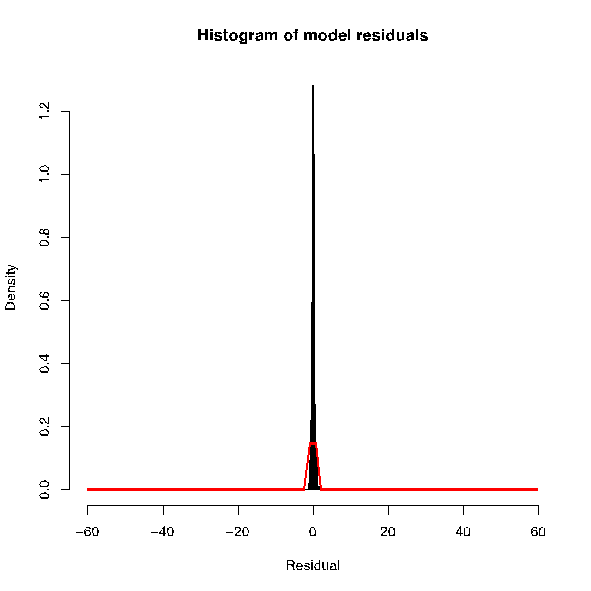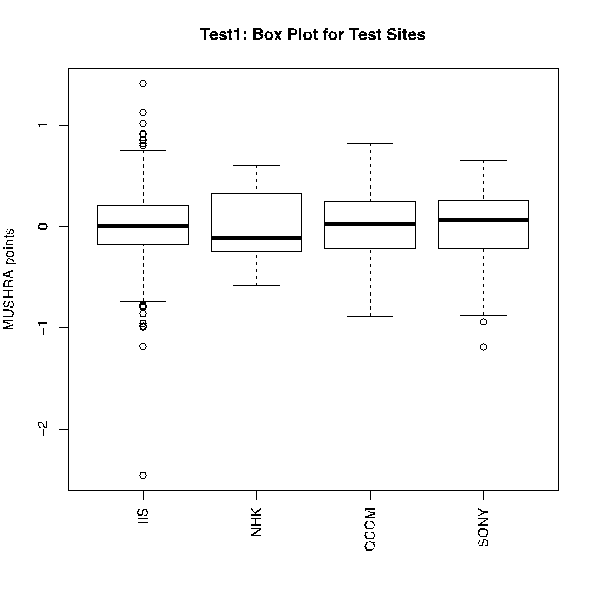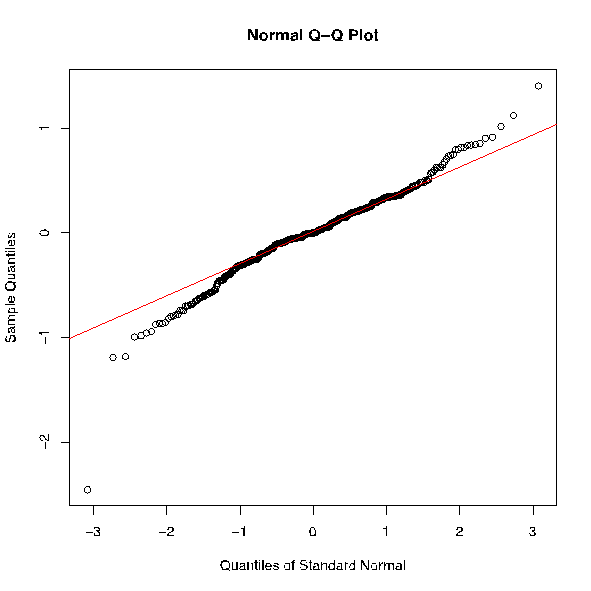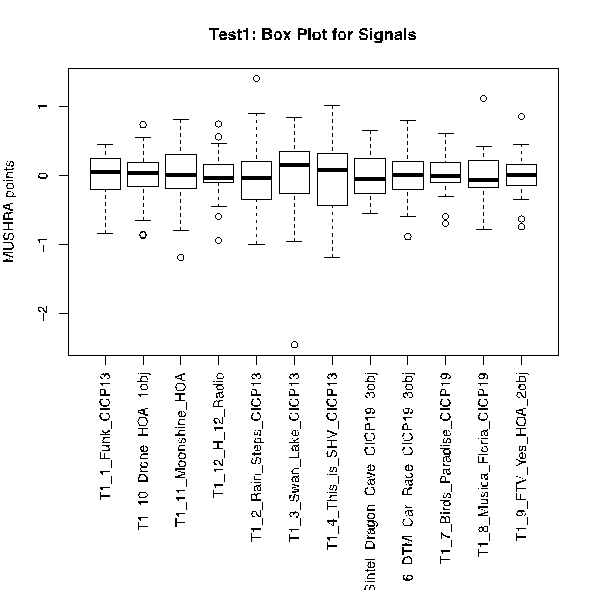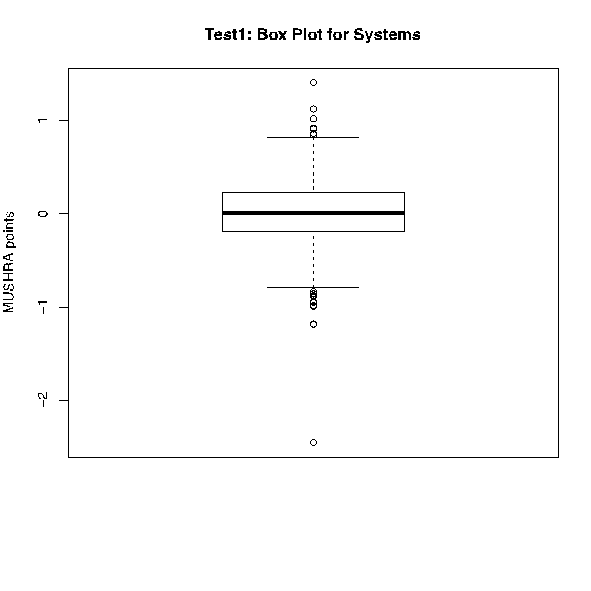Test 2

Model

An aspect of ANOVA is to test the suitability of the model. A simple model incorporating all factors is expressed as:

Score = Lab + System + Signal + Error
The ANOVA report when using this model is:

Df Sum Sq Mean Sq F value Pr(>F)

lab 2 1958 979 14.103 8e-07 ***

sig 11 1217 111 1.594 0.0936 .

sys 5 2837778 567556 8176.454 <2e-16 ***

Residuals 3077 213585 69

---

Signif. codes: 0 ‘***’ 0.001 ‘**’ 0.01 ‘*’ 0.05 ‘.’ 0.1 ‘ ’ 1

The report indicates that model factors lab and sys are highly significant, while factor sig is not significant (at the 5% level of significance).
Performance

Using an ANOVA model does not change the mean score of the system under test. However, because it removes the factor mean effects from the error term, it reduces the error variance and hence the confidence interval on the mean scores. The CI Value (i.e. the ± value used to compute the 95% confidence interval) from ANOVA is

±0.720

In comparison, the average CI from grand mean analysis, as averaged over the systems under test, is

±0.746

Hence, we see that ANOVA gives slightly tighter confidence intervals.

Verification of model assumptions

The following plots verify that the ANOVA residual has approximately a Gaussian distribution, as required for the validity of the ANOVA. Note that the systems Hidden Reference, 7.0 kHz low-pass original and 3.5 kHz low-pass original are removed prior to testing the ANOVA model assumptions since these systems do not get a truly random subjective assessment: subjects are instructed to score the Hidden Reference at 100 and generally tend to score the 7.0 kHz low-pass original and 3.5 kHz low-pass original as some nearly fixed score whose value is based on personal preference.

The left-hand plot below shows a histogram of the Test 2 residual with a best-fit Gaussian distribution (shown in red) superimposed on top. The right-hand plot shows a Normal Q-Q Plot of a Gaussian distribution (red line) and the Test1 residuals. The plot is such that a true Gaussian distribution lies on a straight line. One can see that the Test1 residual deviates from the red line only at the ends, i.e. the tails of the distribution.

Both plots suggest that distribution of the Test 2 residuals are sufficiently close to a Gaussian distribution to apply parametric statistical analysis.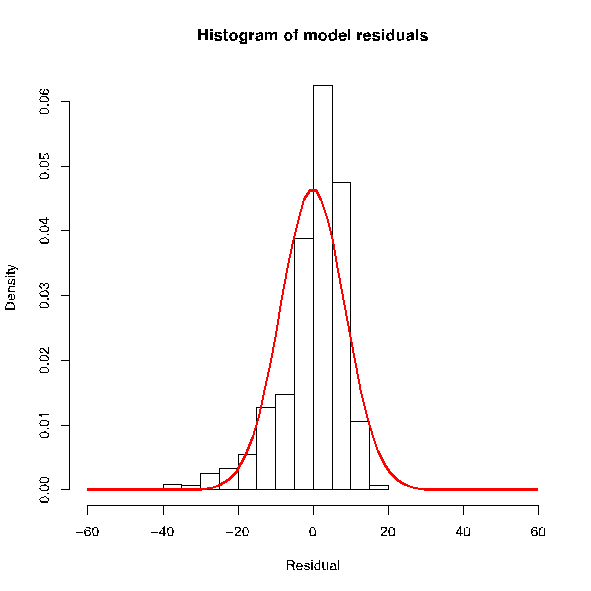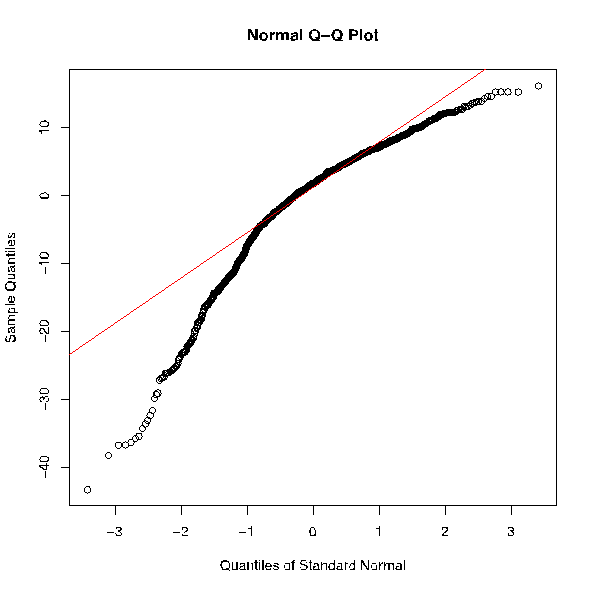The following box plots show the scores associated with each level (or value) of the factors. For each of the factors Lab (Test Site), Test Item (Signals) and System under Test (System), the box plots indicate the distribution of score values after the factor effect is removed. In the box plots:

• The box indicates the range of the middle two quartiles of data (i.e. the box encompasses ±25% of the data, as measured from the mean).

• The “whiskers” indicate ±37.5% of the data, as measured from the mean

• The “circles” indicate data outliers that lie beyond of the ±37.5% region.

The plots indicate that the residuals have the approximately the same distribution for each value of the factor: Test Site and Signal spread is within a few tens of percent while System spread is within a factor of 2. Hence pooling of results from Labs is appropriate.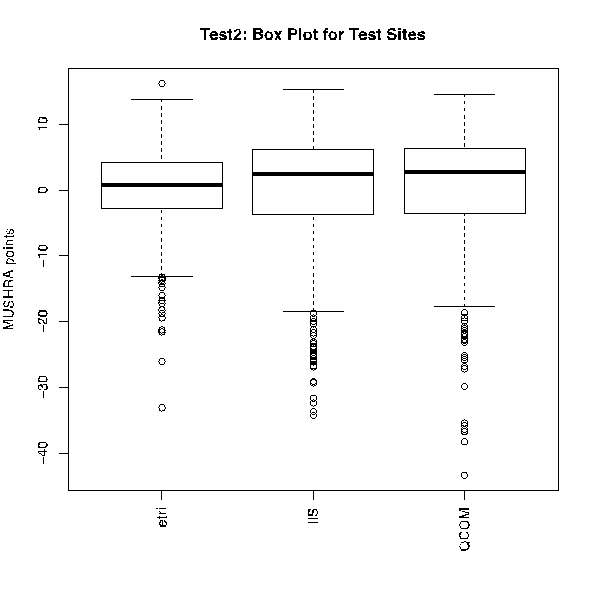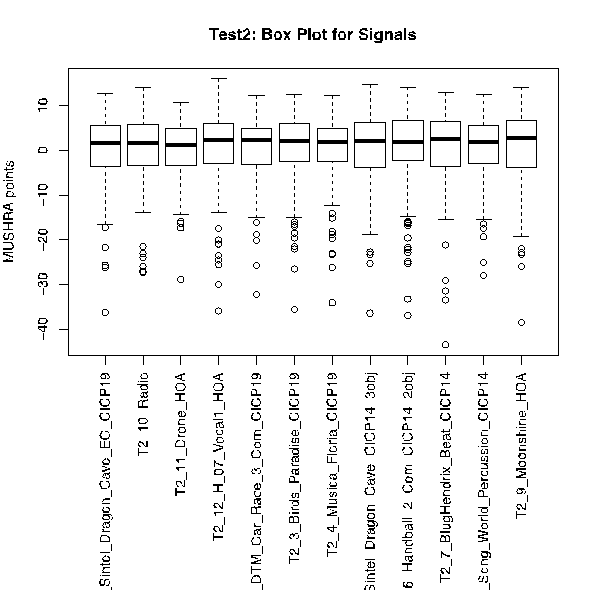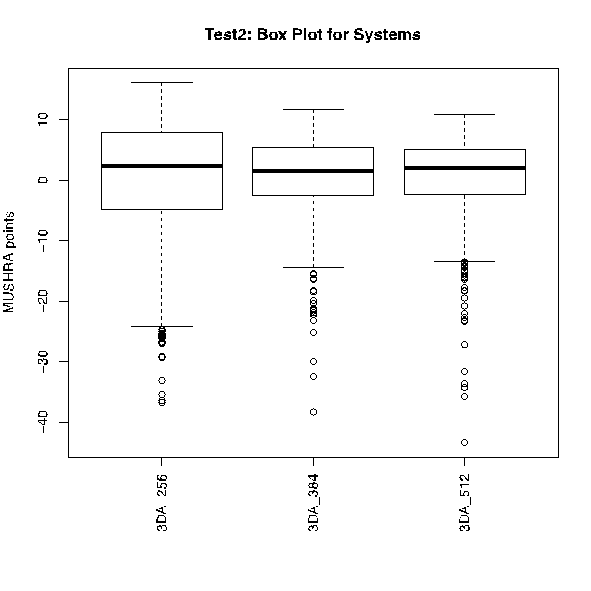Test 3

The structure of Test 3 is similar to that of Test 2, so refer to explanations found in Section “Test 2,” above, for an understanding of the meaning of the following tables and figures.

Model

Df Sum Sq Mean Sq F value Pr(>F)

lab 2 26146 13073 92.530 <2e-16 ***

sig 11 3267 297 2.102 0.0173 *

sys 5 2800774 560155 3964.764 <2e-16 ***

Residuals 3149 444901 141

---

Signif. codes: 0 ‘***’ 0.001 ‘**’ 0.01 ‘*’ 0.05 ‘.’ 0.1 ‘ ’ 1

Performance

ANOVA CI is ±1.015

EXCEL CI is ±1.143
Verification of model assumptions

The histogram of the residual shows is close to having a Gaussian distribution, as shown in the Normal Q-Q plot. Hence it is appropriate to use parametric statistics.

The box plot for Test Sites indicate that the residual variance is approximately the same (within a factor of 2 or 3) for each value of the factor. Hence pooling of results from Test Labs is appropriate.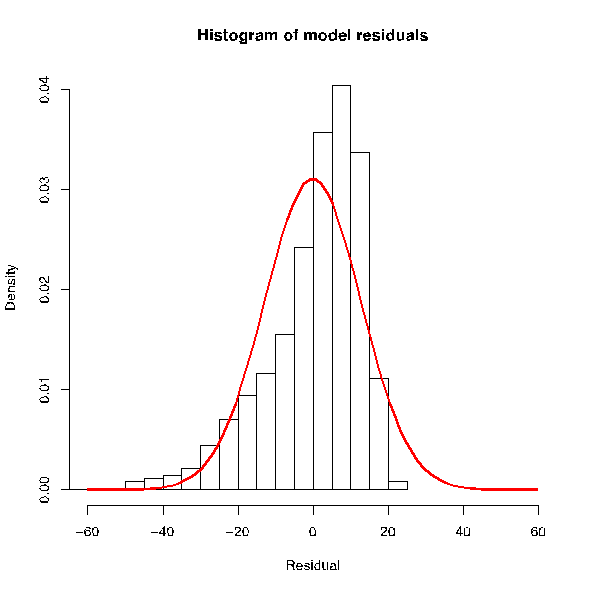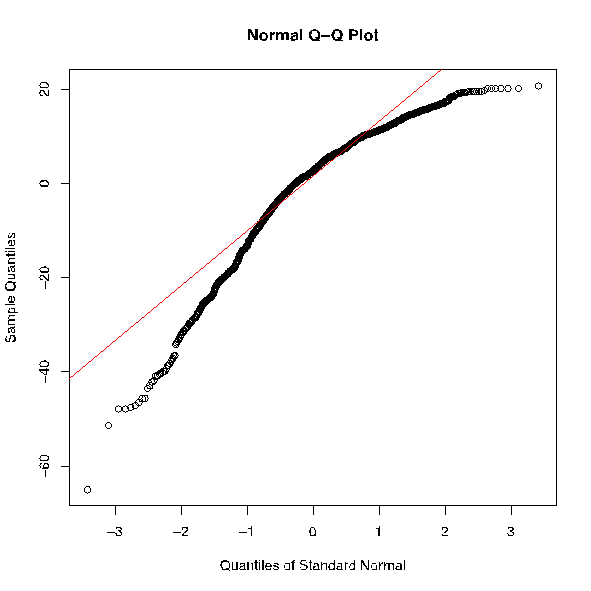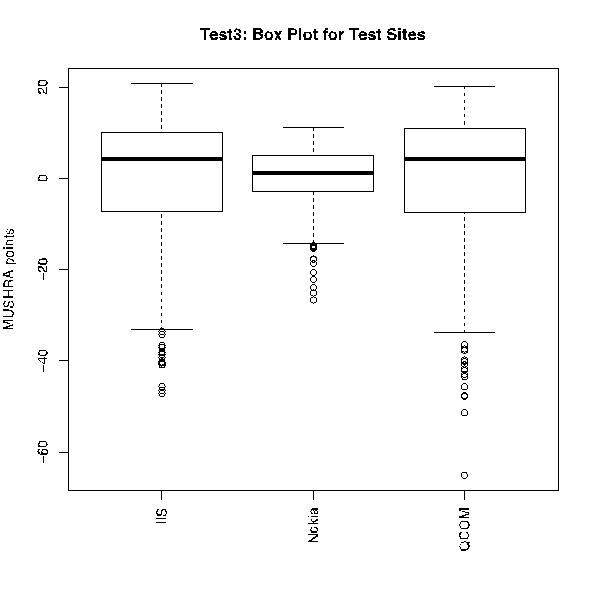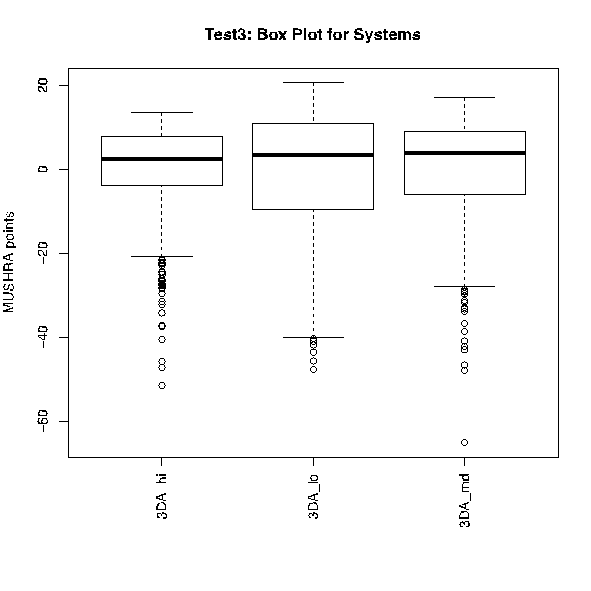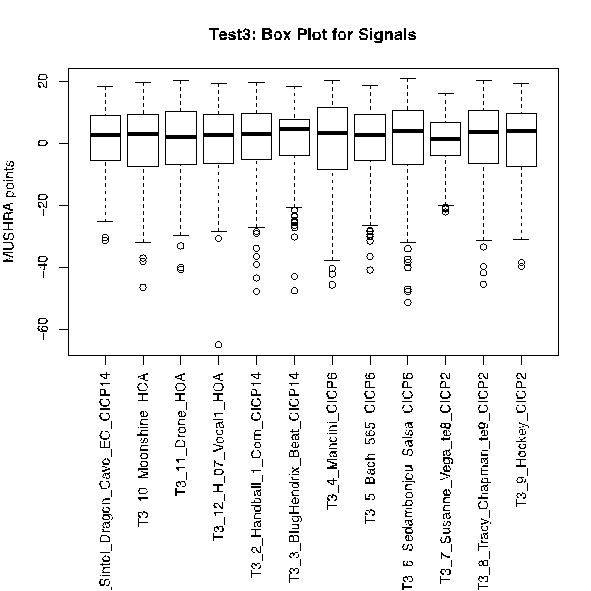Test 4

The structure of Test 4 is similar to that of Test 2, so refer to explanations found in Section “Test 2,” above, for an understanding of the meaning of the following tables and figures.

Model

Df Sum Sq Mean Sq F value Pr(>F)

lab 4 5553 1388 15.490 1.48e-12 ***

sig 11 4997 454 5.068 6.68e-08 ***

sys 3 3610396 1203465 13427.473 < 2e-16 ***

Residuals 3245 290840 90

---

Signif. codes: 0 ‘***’ 0.001 ‘**’ 0.01 ‘*’ 0.05 ‘.’ 0.1 ‘ ’ 1

Performance

ANOVA CI is 0.650

EXCEL CI is 0.586
Verification of model assumptions

The histogram of the residual shows is close to having a Gaussian distribution, as shown in the Normal Q-Q plot. Hence it is appropriate to use parametric statistics.

The box plot for Test Sites indicate that the residual variance is approximately the same (within a factor of 4) for each value of the factor. Hence pooling of results from Labs is appropriate.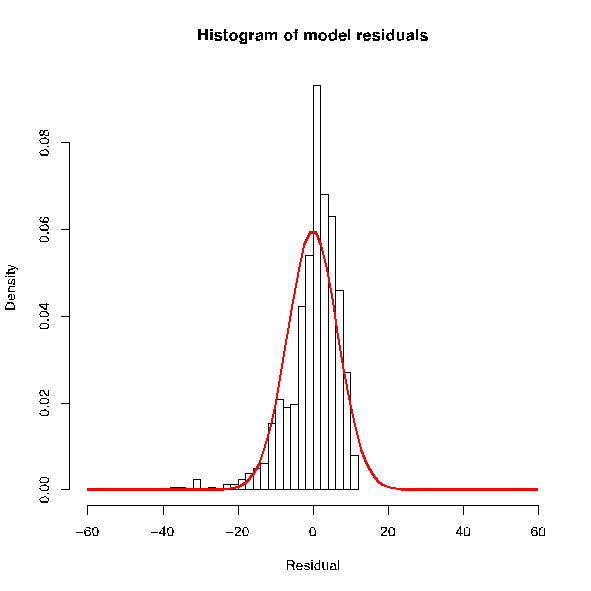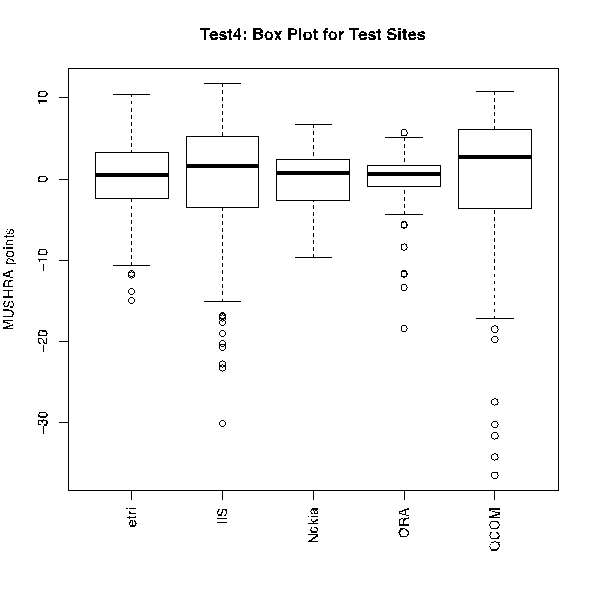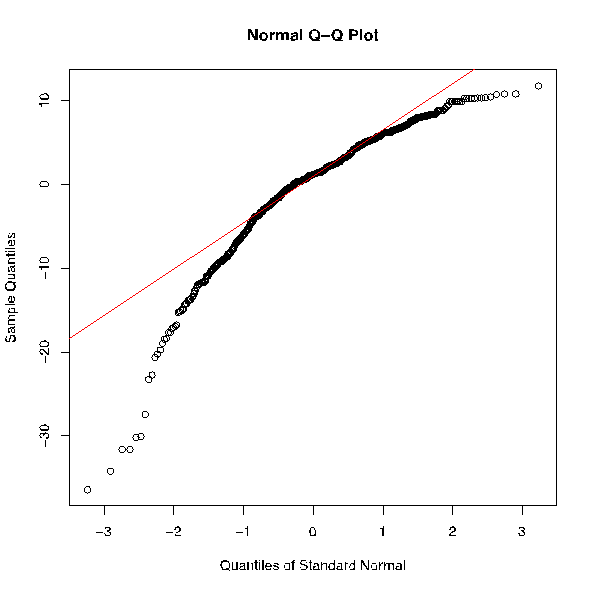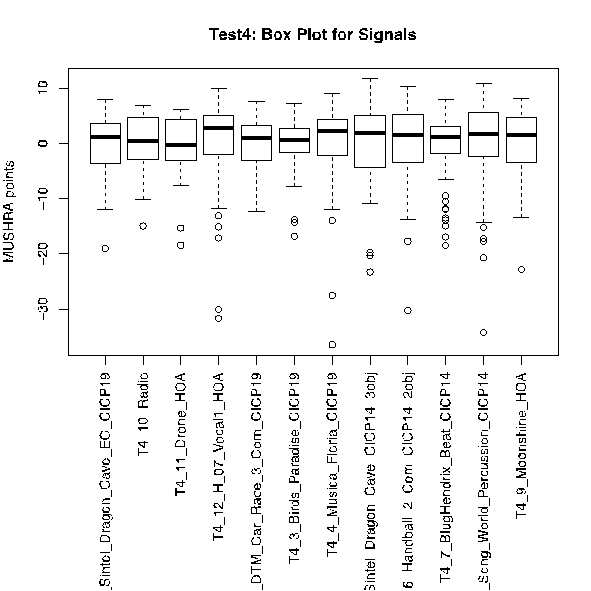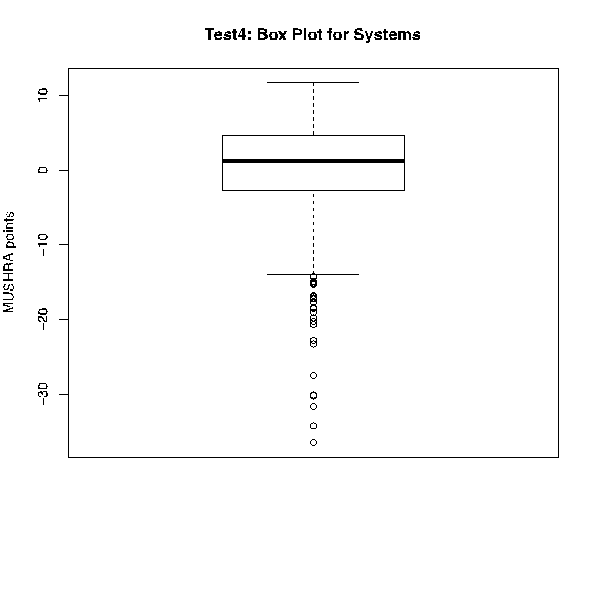References

1. Montgomery, D.C. Design and Analysis of Experiments. John Wiley and Sons, New York, 1976.

2. Bech, S. and Sacharov, N. Perceptual Audio Evaluation, Theory, Method and Application. John Wiley and Sons, Chinchester, West Sussex, England, 2002.

3. Venables, W. N. and Ripley, D. B. Modern Applied Statistics with S, Fourth Edition. Springer, New York, 2002.

4. The R Project for Statistical Computing, http://www.r-project.org/